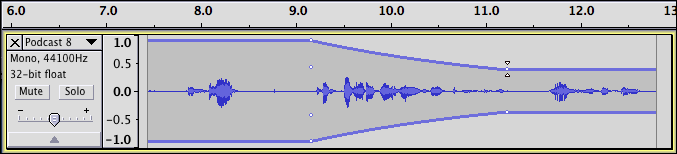# Chord generator

im sorry i ask too much question but i do really like to know & learn at the same time more about music synthesize

``````    (setq note (scale amp (highpass8 (lowpass8 note freq) freq)))))
``````

the above code,in layman’s term, means that this code will divide the pizza into 8 parts ? i think

``````(snd-max(findnote s 440.00 width amplify) maxlength)))
``````

what is the flow of this code.?
from my point of view, from the inner part… findnote has : s = note, 440 = freq, width = q, amplify = amp… the mathematical formula for this part would be… sinA( 2pi f/ samples) ?? since “s” has pass through many filters in one cycle , maxlength the no. of samples limit, sinA since snd-max describes as to compute the max value of amplitude…

what other related formula into this code?.. hoping to do math on each code

“lowpass8” is a low-pass filter
"highpass8 is a high-pass filter
(both are documented in the manual).

``````    (setq note (scale amp (highpass8 (lowpass8 note freq) freq)))))
``````

Working from the innermost “()”
(lowpass8 note freq) … “note” is a sound. This is processed through a low-pass filter at frequency “freq” (a number)

(highpass8 sound freq))… “sound” is the result of the above and is processed through a high-pass filter at frequency “freq”.

(scale amp sound) … scales the sound - it amplifies it by the amount set by “amp” (a number)

(setq note sound) … stets the variable “note” to sound

``````(snd-max(findnote s 440.00 width amplify) maxlength)))
``````

“findnote” is a function that has been created in the code earlier: (defun findnote (note freq q amp)…

“s”, “440.00”, “width” and “amplify” are the variables that are sent to the function. In the function they correspond to the variables “note”, “freq”, “q” and “amp”.

“s” is a special variable in Audacity - it is the sound that is passed to Nyquist from the selected track.

how the extensive is the envelope tool in audacity?

i would like to add a code /function to envelope the sound wave without highlighting or user interventionanalyzing from initial time to last time
refer to the picture above, at 9sec is the initial time to calculate and the ending time is 11… is it possible?

I’m not sure exactly what you are asking, but you could edit the Audacity Project file (.aup) manually in a text editor to create or modify envelopes.

The AUP file is written in XML.
Here is a bit of code from a project file that shows an envelope with two control points, one at time = 9 seconds (value 1.0) and another at time = 11 seconds (value = 0.5).

``````<envelope numpoints="2">
<controlpoint t="9.000000000000" val="1.000000000000"/>
<controlpoint t="11.000000000000" val="0.500000000000"/>
</envelope>
``````

i want to envelope the wave… and calculate using the previous code with the help of pwl…
or i say… everytime i click the plug-in the audacity automatically highlight the wave track as show in the figure i have to set my time where do i start and where do i end then calculate breakpoints…

another approach would be, the audacity will perform the auto-search the peak amplitude of a wave to give me a note.
it is more likely a plot spectrum approach as long as their is a sound it will test all the amplitude then pick the highest one

this is from what i imagine code.

``````    ;; start of function
Pwl  envelope (initial time, last time)
(defun findnote (note freq q amp)
(dotimes (n q note)
(setq note (scale amp (highpass8 (lowpass8 note freq) freq)))))

(setq width 6)
(setq amplify 2.0)
(setq maxlength 30000)

(setq A (snd-max (findnote s 440.00 width amplify) maxlength))
(setq B (snd-max (findnote s 493.88 width amplify) maxlength))
(setq C (snd-max (findnote s 523.25 width amplify) maxlength))
(setq D (snd-max (findnote s 587.33 width amplify) maxlength))
(setq E (snd-max (findnote s 659.26 width amplify) maxlength))
(setq F (snd-max (findnote s 698.46 width amplify) maxlength))
(setq G (snd-max (findnote s 783.99 width amplify) maxlength))

(if (and (> A B)(> A C)(> A D)(> A E)(> A F)(> A G))(print "A")
(if (and (> B A)(> B C)(> B D)(> B E)(> B F)(> B G))(print "B")
(if (and (> C A)(> C B)(> C D)(> C E)(> C F)(> C G))(print "C")
(if (and (> D A)(> D B)(> D C)(> D E)(> D F)(> D G))(print "D")
(if (and (> E A)(> E B)(> E C)(> E D)(> E F)(> E G))(print "E")
(if (and (> F A)(> F B)(> F C)(> F D)(> F E)(> F G))(print "F")
(if (and (> G A)(> G B)(> G C)(> G D)(> G E)(> G F))(print "G")